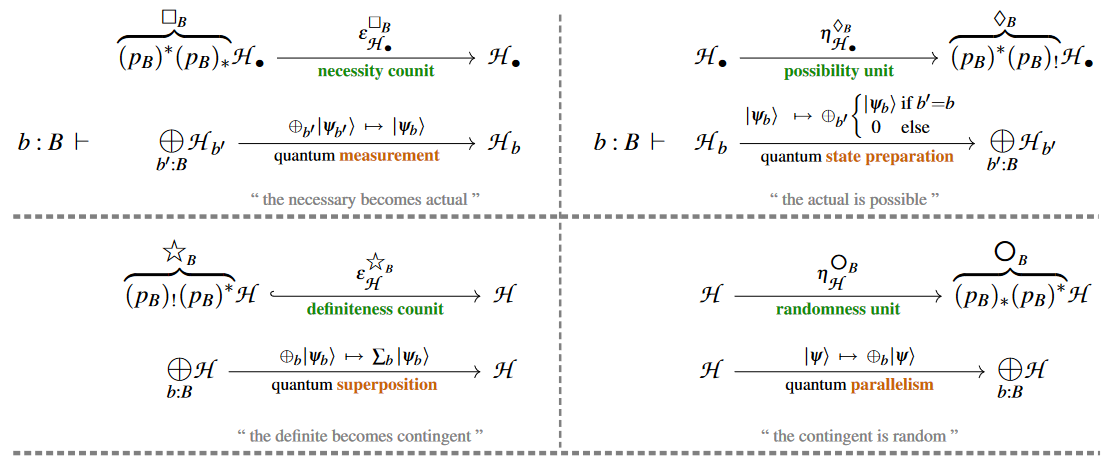# nLab Kleisli category of a comonad

Contents

### Context

#### 2-Category theory

2-category theory

Definitions

Transfors between 2-categories

Morphisms in 2-categories

Structures in 2-categories

Limits in 2-categories

Structures on 2-categories

#### Higher algebra

higher algebra

universal algebra

# Contents

## Idea

Formally dually to how a monad has a Kleisli category so also a comonad $P \colon \mathcal{C}\to\mathcal{C}$ has a Kleisli category: its objects are the objects of $\mathcal{C}$, a morphism $f \colon c_1 \to c_2$ in the Kleisli category is a morphism

$\tilde f \colon P(c_1) \longrightarrow c_2$

in $\mathcal{C}$, and the composition of two such in the Kleisli category is represented by the morphism in $\mathcal{C}$ given by

$\widetilde{f_2 \circ f_1} \colon P(c_1) \longrightarrow P(P(c_1)) \stackrel{P(\tilde f_1)}{\longrightarrow} P(c_2) \stackrel{\tilde f_2}{\longrightarrow} c_3 \,.$

Sometimes the term co-Kleisli category is encountered, but this is redundant, since there is only one notion of Kleisli category for a comonad.

## Examples

### General

###### Example

For an idempotent comonad the Kleisli category is the coreflective subcategory of its modal types.

###### Example

In typed functional programming, the Kleisli category of a comonad can be used to model call-by-name? programming languages. Dually, the Kleisli category of a monad may be used to model call-by-value?-programming, see there.

Generally, see at monad (in computer science) for more on this.

### Specific

###### Example

(matrix multiplication as Kleisli composition)
For

• $k$ a field

• $B$ be a finite set

consider the base change adjoint triple of $B$-indexed sets of $k$-vector spaces ($k$-vector bundles over $B$):

By the assumption that $B$ is finite and since Vect has biproducts, the colimit/coproduct

$(p_B)_! \mathscr{V}_\bullet \;=\; \coprod_{b : B} \mathscr{V}_b$

and the limit/product

$(p_B)_\ast \mathscr{V}_\bullet \;=\; \prod_{b : B} \mathscr{V}_b$

agree (we have an ambidextrous adjunction) and are both equivalent to the direct sum:

$(p_B)_! \mathscr{V}_\bullet \;\simeq\; (p_B)_\ast \mathscr{V}_\bullet \;=\; \underset{b \colon B}{\bigoplus} \mathcal{V}_b \,.$

Accordingly, the underlying endofunctors of the induced monad and comonad on $k Vec_B$

$\Box_B \;\coloneqq\; (p_B)^\ast (p_B)_\ast \;\;\vdash\;\; \lozenge_B \;\coloneqq\; (p_B)^\ast (p_B)_! \;\;\colon\;\; k Vec_B \longrightarrow k Vec_B$

agree, to make a self-adjoint functor

$(p_B)^\ast \bigotimes_B \;\;\vdash\;\; (p_B)^\ast \bigotimes_B \;\;\;\colon\;\;\; k Vec \longrightarrow k Vec$

which carries the structure both of a monad and of a comonad.

We claim that

1. the categories of

1. free $(p_B)^\ast \oplus_B$-algebras

2. co-free $(p_B)^\ast \oplus_B$-coalgebras

are both equivalent to the category $k Vec$ of $k$-vector spaces,

2. where:

1. the Kleisli category of $\bigoplus_B$ exhibits such linear maps as $B$-tuples of columns of $B \times B$-block matrices;

2. the coKleisli category of $\bigoplus_B$ exhibits such linear maps as $B$-tuples of rows of $B \times B$-block matrices

with (co)Kleisli composition expressing the operation of block-matrix multiplication.

In particular, on the (co)free algebras of the form $\bigotimes_B (p_B)^\ast k$ this statement holds in the sense of actual $B \times B$-matrices with entries in $k$ (as opposed to block matrices).

Since every linear map admits some $B \times B$-block matrix representation, the second statement implies the first by the Kleisli theorem (see here).

Moreover, the second statement follows by direct inspection of the Kleisli morphisms (and dually for the coKleisli morphisms):

For this, identify a coKleisli morphism (where our notation now borrows from dependent linear type theory in order to express morphisms between $B$-indexed sets of vector spaces)

$b \colon B \;\; \vdash \;\; \Big( \underset{b' \colon B}{\bigoplus} \mathscr{V}_{b'} \Big) \xrightarrow{\; F_b \;} \mathscr{W}_b$

with the $B$-tuple of “block $B \times 1$” matrices $(F_b)_{b \colon B}$ that it evidently encodes.

Observe that the comultiplication on the $\bigotimes_B$-comonad is given by the diagonal map into the biproduct, coming from the “randomness unit” (terminology from modal quantum logic, following CQTS (2022)):Using this, one readily sees that the Kleisli construction indeed sends such $B$-tuples $(F_b)_{b \colon B}$ of block-matrix rows to the linear map $F$ which is given by the corresponding full matrix, and that the Kleisli composite with any other

$b \colon B \;\; \vdash \;\; \Big( \underset{b' \colon B}{\bigoplus} \mathscr{W}_{b'} \Big) \xrightarrow{\; G_b \;} \mathscr{Z}_b$

is indeed the row-decomposition of the corresponding matrix product:

The formally dual argument (now using the “definiteness counit”) shows the analogous statement for Kleisli morphisms and column-decomposition of block matrices:

###### Example

For $P= Jet$ a jet comonad, then morphisms in its coKleisli category are differential operators (see there).

The equivalence of categories between the Kleisli category over a given comonad with the Kleisli category of an adjoint monad (if it exists):

Some introductory material on comonads, coalgebras and co-Kleisli morphisms:

• Paolo Perrone, Notes on Category Theory with examples from basic mathematics, Chapter 5. (arXiv)